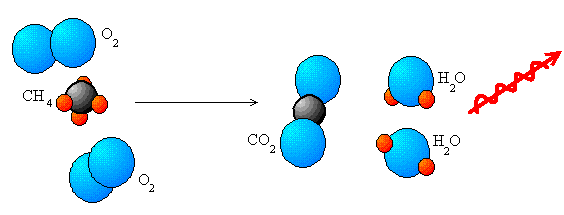## Types of Redox Reactions

#### Learning Objective

• Explain the processes involved in a redox reaction and describe what happens to their various components.

#### Key Points

• In combination reactions, two elements are combined. $A + B \rightarrow AB$.
• In decomposition reactions, a compound is broken down into its constituent parts. $AB \rightarrow A + B$ .
• In displacement reactions, one or more atoms is swapped out for another. $AB + C \rightarrow A + CB$ .
• In combustion reactions, a compound reacts with oxygen to produce carbon dioxide, water, and heat.
• In disproportionation reactions, a molecule is both reduced and oxidized; these types of reactions are rare.

#### Terms

• combustiona process in which a fuel combines with oxygen, usually at high temperature, releasing CO2, H2O, and heat
• redoxa shorthand term for “reduction-oxidation,” two methods of electron transfer that always occur together

Redox reactions are all around us. In fact, much of our technology, from fire to laptop batteries, is largely based on redox reactions. Redox (reduction-oxidation) reactions are those in which the oxidation states of the reactants change. This occurs because in such reactions, electrons are always transferred between species. Redox reactions take place through either a simple process, such as the burning of carbon in oxygen to yield carbon dioxide (CO2), or a more complex process such as the oxidation of glucose (C6H12O6) in the human body through a series of electron transfer processes.

The term “redox” comes from two concepts involved with electron transfer: reduction and oxidation. These processes are defined as follows:

• Oxidation is the loss of electrons or an increase in oxidation state by a molecule, atom, or ion.
• Reduction is the gain of electrons or a decrease in oxidation state by a molecule, atom, or ion.

A simple mnemonic for remembering these processes is “OIL RIG”—Oxidation Is Losing (electrons), Reduction Is Gaining (electrons).

Redox reactions are matched sets: if one species is oxidized in a reaction, another must be reduced. Keep this in mind as we look at the five main types of redox reactions: combination, decomposition, displacement, combustion, and disproportion.

## Combination

Combination reactions “combine” elements to form a chemical compound. As usual, oxidation and reduction occur together.

General equation:  $A + B \rightarrow AB$

Sample 1. equation: 2 H2 + O2 → 2 H2O

The sum of oxidation states in the reactants is equal to that in the products: 0 + 0 → (2)(+1) + (-2)

In this equation, both H2 and O2 are the molecular forms of their respective elements and therefore their oxidation states are 0. The product is H2O: the oxidation state is -2 for oxygen and +1 for hydrogen.

## Decomposition

Decomposition reactions are the reverse of combination reactions, meaning they are the breakdown of a chemical compound into its component elements.

General equation: AB → A + B

Sample 2. equation: 2 H2O → 2 H2 + O2

Calculation: (2)(+1) + (-2) = 0 → 0 + 0

In this equation, the water is “decomposed” into hydrogen and oxygen, both of which are neutral. Similar to the previous example, H2O has a total oxidation state of 0, with each H taking on a +1 state and the O a -2; thus, decomposition oxidizes oxygen from -2 to 0 and reduces hydrogen from +1 to 0.

## Displacement

Displacement reactions, also known as replacement reactions, involve compounds and the “replacing” of elements. They occur as single and double replacement reactions.

General equation (single displacement): A + BC → AB + CA

A single replacement reaction “replaces” an element in the reactants with another element in the products.

Sample 3. equation: Cl2 + 2 NaBr → 2 NaCl + Br2

Calculation: 0 + [(+1) + (-1) = 0] $\rightarrow$ [(+1) + (-1) = 0] + 0

In this equation, Cl is reduced and replaces Br, while Br is oxidized.

General equation (double displacement): AB + CD → AD + CB

A double replacement reaction is similar to a single replacement reaction, but involves “replacing” two elements in the reactants with two in the products.

Sample 4. equation: Fe2O3 + 6 HCl → 2 FeCl3 + 3 H2O

In this equation, Fe and H as well as O and Cl trade places.

## Combustion

Combustion reactions always involve oxygen and an organic fuel. In the following image, we see methane combusting to release energy.Combustion reaction of methaneThis is an example of a combustion reaction, a redox process. Methane ($\text{CH}_4$), reacts with oxygen ($\text{O}_2$)  to form carbon dioxide ($\text{CO}_2$) and two water molecules ($2\text{H}_2\text{O}$).

The general equation of a combustion reaction is:

$\text{C}_x\text{H}_y +\left( x + \dfrac{y}{4} \right) \text{O}_2 \rightarrow x \text{CO}_2 + \dfrac{y}{2}\text{H}_2\text{O}$

## Disproportionation

In some redox reactions, substances can be both oxidized and reduced. These are known as disproportionation reactions. One real-life example of such a process is the reaction of hydrogen peroxide, H2O2, when it is poured over a wound. At first, this might look like a simple decomposition reaction, because hydrogen peroxide breaks down to produce oxygen and water:

2 H2O2(aq) → 2 H2O(l) + O2(g)

The key to this reaction lies in the oxidation states of oxygen, however. Notice that oxygen is present in the reactant and both products. In H2O2, oxygen has an oxidation state of -1. In H2O, its oxidation state is -2, and it has been reduced. In O2 however, its oxidation state is 0, and it has been oxidized. Oxygen has been both oxidized and reduced in the reaction, making this a disproportionation reaction. The general form for this reaction is as follows:

2A → A’ + A”